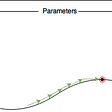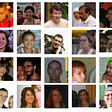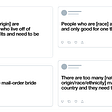# WSDM KKBox’s Music Recommendation Challenge

1. KKBOX It is Asia's leading music streaming service, holding the world’s most comprehensive Asia-pop Music library with over 40 million tracks
2. Offers a generous, unlimited version of their service to millions of people supported by advertising and paid subscriptions.
3. Working on a Freemium basis, both “pay-per-month” buyers or free service listeners on smartphones, TV, media centre and computer
4. The services are mainly targeting the music market of southeast Asia, Focusing on regions including Taiwan, Hong Kong, Malaysia, Singapore etc.
5. The Internet made life easy in terms of selecting music of users’ choice, but still, algorithms are needed to recommend favourite music to users without selecting manually.
6. Building a recommendation system based on top features of the dataset and using similarity measures across them to predict the list of top tracks recommended for the users.

Objective:

we have to predict the chances of a user listening to a song repetitively after the first observable listening event within a time window was triggered.

Overview:

Data:- The dataset is available on https://www.kaggle.com/c/kkbox-music-recommendation-challenge.

Table of content:

2. Data
3. EDA
4. Feature Engineering
5. Data Preprocessing
6. Models
7. Comparison
8. Conclusion & Future work
9. References

Introduction :

Music is the next dimension of connection when it comes to expressing your feelings. Music helps anybody to connect with what you are doing. It elevates mood and rejuvenates the waves of thoughts Different people have different flavours of music. Music has served its users with various platforms like waves of a culture of Cassette, Walkman era, i-pods, FM-Radios and now the latest musical apps like Spotify, Amazon Prime Music, Deezer, SoundCloud, Gaana, etc.

The Internet made life easy in terms of selecting music of users’ choice, but still, algorithms are needed to recommend favourite music to users without selecting manually. Thereby making it user friendly for the user and increasing business for the organizations

# 1. Business Problem and constraints:

WSDM (International Conference on Web Search and Data Mining) has given a challenge to the Kaggle community to build a better music recommendation system using a donated dataset from KKBOX.

Given a set of features, we have to predict whether the user would like to listen to the recommended song or not.

1. Song recommendation should not take hours or days. A few minutes/seconds would be sufficient to predict the chances of listening.
3. Prediction should be interpretable.
• ML Problem Formulation

We have to build the model which will predict whether a user will re-listen to the song by evaluating given features of the user and songs. We can convert this problem into a classification problem and can apply various classification algorithms.

# 2. Data

Total 5 data files are given:

train.csv: this file includes

user_id (msno),

song_id,

source_system_tab (where the event was triggered),

source_type (an entry point a user first plays music),

source_screen_name (name of the layout user sees),

target (​ 1 means there is a recurring listening event(s) triggered within a month after the user’s a very first observable listening event, target=0​ otherwise ).

test.csv: Contains fields same as above except target, which we have to predict.

songs.csv: It includes fields like

song_id,

song_length,

genre_id,

artist_name,

composer,

lyricist

language.

members.csv: It contains attributes like

msno (user_id),

city,

bd ,

gender,

register_via (register method),

register_init_time (date),

expirartion_date (date).

song_extra_info.csv: This file contains

song_id,

song_name

ISRC (International Standard Recording Code) is used to identify songs.

# 3. EDA:

Let’s explore our data and understand the behaviour of each and every feature with plots.

I. Train features:

We have count plots for source_type, source_system_tab and source_screen_name. We can see from the plots that all our features are almost balanced with respect to class labels in each value of the feature.

II. song features:

We have different types of languages in songs data which are denoted by numbers. We can see that most users prefer listening to songs from ‘-1’ and ‘52’ languages.

Most of the users prefer registration via ‘4’,’7' and ‘9’ methods.

III. members data:

From the above PDFs we can say that after 2012, people started registering themselves for listening to music, hence their expiration periods is also found to be near to 2020.

1. we can see the various artist label is the majority.
2. then we have echo music, Billie holiday, billy Vaughan having most of the search.
3. Heart, love time remix, a feat many more are the key drivers when it comes to search.

# 4. Feature Engineering:

we will remove those features which are having more than 25% missing values and start feature Engineering. We will also fill in missing values according to features.

`def filling_missing_values(data):         data['source_system_tab'].fillna('no_system_tab', inplace=True)  data['source_screen_name'].fillna('no_screen_name', inplace=True)  data['source_type'].fillna('np_source_type', inplace=True)  data['bd'].fillna(0, inplace=True)  data['gender'].fillna('gender_missing', inplace=True)  data['song_length'].fillna(0, inplace=True)  data['genre_ids'].fillna(0, inplace=True)  data['artist_name'].fillna('no_artist_name', inplace=True)  data['language'].fillna('no_language', inplace=True)  data['name'].fillna('no_name', inplace=True)  return data`

Members have registration and expiration dates, from which we can extract features like membership time, individual day, month and year.

`def extract_date_fatures(data): # convert into date format        data['expiration_date']=pd.to_datetime(data['expiration_date'],format='%Y%m%d')data['registration_init_time']=pd.to_datetime(data['registration_init_time'], format='%Y%m%d') # get membership period from registration and expiration datesdata['membership_days']=data['expiration_date'].subtract(data['registration_init_time']).dt.days.astype(int)# extract year, month and day from datesdata['registration_year']=data['registration_init_time'].dt.yeardata['registration_month'] = data['registration_init_time'].dt.monthdata['registration_day'] = data['registration_init_time'].dt.daydata['expiration_year'] = data['expiration_date'].dt.yeardata['expiration_month'] = data['expiration_date'].dt.monthdata['expiration_day'] = data['expiration_date'].dt.dayreturn data`
• We will extract individual features independent from members, songs and songs_extra. After merging all files we will extract group-by features.
• We will filter the age between 0 and 75.
`def filter_age(x):if x >= 0 and x <= 75:  return xelse:  return np.nan`
• We will extract genre_id_count, artist_count from genre_id and artist. Some songs have many artists and genres so we will also extract the first artist name and first genre_id.
`def generate_genre_ids(data):    '''Function to sepearate each genre_id and count total number of genre_ids'''    genre_ids_matrix = np.zeros((data.shape, 4))for i in range(data.shape):        ids = str(data['genre_ids'].values[i]).split('|')        if len(ids) > 2:            genre_ids_matrix[i, 0] = (ids)            genre_ids_matrix[i, 1] = (ids)            genre_ids_matrix[i, 2] = (ids)        elif len(ids) > 1:            genre_ids_matrix[i, 0] = (ids)            genre_ids_matrix[i, 1] = (ids)        elif len(ids) == 1:            genre_ids_matrix[i, 0] = (ids)        genre_ids_matrix[i, 3] = len(ids)data['first_genre_id'] = genre_ids_matrix[:, 0] # keeps first genre_id    data['second_genre_id'] = genre_ids_matrix[:, 1] # keeps second genre_id    data['third_genre_id'] = genre_ids_matrix[:, 2] # keeps third genre_id    data['genre_ids_count'] = genre_ids_matrix[:, 3] # keeps count of genre_ids    return data`

the code snippet is for artist features.

`def artist_count(x):    '''Function to count total number of artists for each song'''    return x.count('and') + x.count(',') + x.count(' feat') + x.count('&') + 1def get_first_artist(x):    '''Function to extract first artist name from more than one artists'''    if x.count('and') > 0:        x = x.split('and')    if x.count(',') > 0:        x = x.split(',')    if x.count(' feat') > 0:        x = x.split(' feat')    if x.count('&') > 0:        x = x.split('&')    return x.strip()`
• We will extract `song_year`, `country_code` and `registration_code` from isrc feature.
`def calcualte_songs_features(data):    '''Function to extract features from isrc.'''    isrc = data['isrc']    data['country_code'] = isrc.str.slice(0, 2)    data['registration_code'] = isrc.str.slice(2, 5)    data['song_year'] = isrc.str.slice(5, 7).astype(float)    data['song_year'] = data['song_year'].apply(lambda x: 2000+x if x < 18 else 1900+x)    data['isrc_missing'] = (data['country_code'] == 0) * 1.0    return data`

group by feature to group all similar tastes and likingness

`def groupby(data):    member_song_count = data.groupby('msno').count()['song_id'].to_dict()    data['member_song_count'] = data['msno'].apply(lambda x: member_song_count[x])artist_song_count = data.groupby('first_artist_name').count()['song_id'].to_dict()    data['artist_song_count'] = data['first_artist_name'].apply(lambda x: artist_song_count[x])composer_song_count = data.groupby('first_composer').count()['song_id'].to_dict()    data['composer_song_count'] = data['first_composer'].apply(lambda x: composer_song_count[x])lyricist_song_count = data.groupby('first_lyricist').count()['song_id'].to_dict()    data['lyricist_song_count'] = data['first_lyricist'].apply(lambda x: lyricist_song_count[x])first_genre_id_song_count = data.groupby('first_genre_id').count()['song_id'].to_dict()    data['genre_song_count'] = data['first_genre_id'].apply(lambda x: first_genre_id_song_count[x])lang_song_count = data.groupby('language').count()['song_id'].to_dict()    data['lang_song_count'] = data['language'].apply(lambda x: lang_song_count[x])song_member_count = data.groupby('song_id').count()['msno'].to_dict()    data['song_member_count'] = data['song_id'].apply(lambda x: song_member_count[x])     return data`

# 5. Data Preprocessing:

After extracting all features from data files it's time to transform all these features. We have numerical and categorical features. There are techniques like Normalization, Standardization. We will use Standardization, as it standardizes features by removing the mean and scaling to unit variance. For categorical features, we have one-hot encoding, Label encoding, Response encoding etc. We will use Label-encoder for our categorical features.

`numeric_features = ['bd','registered_via', 'song_length', 'membership_days','genre_ids_count', 'artist_count','is_featured','lyricist_count','song_lang_boolean',                    'composer_count','song_size_boolean','isrc_missing']for i in numeric_features:    scaler = StandardScaler()    X_train_fe[i] = scaler.fit_transform(X_train_fe[i].values.reshape(-1,1))    X_val_fe[i] = scaler.transform(X_val_fe[i].values.reshape(-1,1))    X_test_fe[i] = scaler.transform(X_test_fe[i].values.reshape(-1,1))cat_features = ['msno', 'song_id', 'source_system_tab', 'source_screen_name', 'source_type', 'city', 'gender',\                'registered_via', 'name', 'registration_year', 'registration_month', 'registration_day',\                'expiration_year', 'expiration_month', 'expiration_day', 'first_genre_id', 'second_genre_id',\                'third_genre_id', 'first_artist_name', 'country_code',                'registration_code','song_year', 'language']for i in cat_features:    enc = LabelEncoder()    print(i)    combined = X_train_fe[i].append(X_val_fe[i])    combined = set(combined.append(X_test_fe[i]))    combined = np.array(list(combined))    enc = enc.fit(combined)    X_train_fe[i] = enc.transform(X_train_fe[i].values.reshape(-1,1))    X_val_fe[i] = enc.transform(X_val_fe[i].values.reshape(-1,1))    X_test_fe[i] = enc.transform(X_test_fe[i].values.reshape(-1,1))`

# 6. Models:

As we have stated earlier that we will pose this problem as a classification problem and we can apply various classification algorithms on top of our data points. We will discuss the feature importance for each model at the end of this section. In the comparison section, we will discuss the results.

1. Logistic Regression

Logistic Regression hyperparameter tuning using GridSearchCV

`# Hyper parameter tuning using GridearchCV for LRparameters = {'penalty':['l2', 'l1'], 'alpha':[10 ** x for x in range(-4, 2)]}clf = SGDClassifier(loss='log', n_jobs=-1, random_state=23, class_weight='balanced' )model = GridSearchCV(clf, parameters, scoring = 'roc_auc', n_jobs=-1, verbose=2, cv=3)model.fit(tr_data, y_train)print(model.best_estimator_)print('train AUC = ',model.score(tr_data, y_train))print('val AUC = ',model.score(val_data, y_cv))`

2. Support Vector Machines :

`# Hyper parameter tuning using GridearchCV for SVMparameters = {'penalty':['l2', 'l1'], 'alpha':[10 ** x for x in range(-4, 2)]}clf = SGDClassifier(loss='hinge', n_jobs=-1, random_state=23, class_weight='balanced' )model = GridSearchCV(clf, parameters, scoring = 'roc_auc', n_jobs=-1, verbose=2, cv=3)model.fit(tr_data, y_train)print(model.best_estimator_)print('train AUC = ',model.score(tr_data, y_train))print('val AUC = ',model.score(val_data, y_cv))`

3. Random Forest :

`start = time.time()parameters = {'n_estimators':[100, 200, 300, 500,1000]}clf = RandomForestClassifier(random_state=23, class_weight='balanced', n_jobs=-1)model = GridSearchCV(clf, parameters, scoring = 'roc_auc', verbose=2, cv=3)model.fit(tr_data, y_train)print(model.best_estimator_)print('train AUC = ',model.score(tr_data, y_train))print('val AUC = ',model.score(val_data, y_cv))print('Time taken for hyper parameter tuning is : ', (time.time() -  start))`

4. Decision Tree :

`start = time.time()parameters = {'max_depth':[3, 5, 8, 10, 15, 50],'min_samples_split':[5, 10, 100, 500, 1000],'max_leaf_nodes': list(range(2, 100))}clf = DecisionTreeClassifier(random_state=23, class_weight='balanced' )model = GridSearchCV(clf, parameters, scoring = 'roc_auc', n_jobs=-1, verbose=2, cv=3)model.fit(tr_data, y_train)print(model.best_estimator_)print('train AUC = ',model.score(tr_data, y_train))print('val AUC = ',model.score(val_data, y_cv))print('Time taken for hyper parameter tuning is : ', (time.time() -  start))`

5. GBDT :

`GBDT =  GradientBoostingClassifier()parameters = {'max_depth' : [5,10,50], 'n_estimators' : [5,100,500]}clf = GridSearchCV(GBDT,parameters,scoring = 'roc_auc',verbose=10,return_train_score=True )gs = clf.fit(tr_data, y_train)print("Best Params : " , gs.best_params_)print("Best Score : " , gs.best_score_)`

`adb = AdaBoostClassifier()parameters = {'n_estimators' : [1000,1100,1200,1300]}clf = GridSearchCV(adb, parameters,scoring = 'roc_auc')res = clf.fit(tr_data, y_train)print("Best Params : " , res.best_params_)print("Best Score : " , res.best_score_)`

7. Light GBM:

`#https://www.analyticsvidhya.com/blog/2017/06/which-algorithm-takes-the-crown-light-gbm-vs-xgboost/params = {        'objective': 'binary',        'metric': 'binary_logloss',        'boosting': 'gbdt',        'learning_rate': 0.3 ,        'verbose': 0,        'num_leaves': 108,        'bagging_fraction': 0.95,        'bagging_freq': 1,        'bagging_seed': 1,        'feature_fraction': 0.9,        'feature_fraction_seed': 1,        'max_bin': 256,        'max_depth': 10,        'num_rounds': 400,        'metric' : 'auc'    }`

8. Deep Learning with embedding layer:

`cat_vars = ['msno', 'song_id', 'source_system_tab', 'source_screen_name', 'source_type', 'name','expiration_year', 'first_artist_name','registration_code','song_year', 'language']cat_sizes = {}cat_embsizes = {}for cat in cat_vars:    cat_sizes[cat] = tr_data[cat].nunique()    cat_embsizes[cat] = min(50, cat_sizes[cat]//2+1)    input1 = Input(shape=(1,))x1 = Embedding(input_dim=cat_sizes['msno']+1, output_dim=cat_embsizes['msno'],trainable=True)(input1)x1 = Flatten()(x1)input2 = Input(shape=(1,))x2 = Embedding(input_dim=cat_sizes['song_id']+1, output_dim=cat_embsizes['song_id'],trainable=True)(input2)x2 = Flatten()(x2)input3 = Input(shape=(1,))x3 = Embedding(input_dim=cat_sizes['source_system_tab']+1, output_dim=cat_embsizes['source_system_tab'],trainable=True)(input3)x3 = Flatten()(x3)input4 = Input(shape=(1,))x4 = Embedding(input_dim=cat_sizes['source_screen_name']+1, output_dim=cat_embsizes['source_screen_name'],trainable=True)(input4)x4 = Flatten()(x4)input5 = Input(shape=(1,))x5 = Embedding(input_dim=cat_sizes['source_type']+1, output_dim=cat_embsizes['source_type'],trainable=True)(input5)x5 = Flatten()(x5)input6 = Input(shape=(1,))x6 = Embedding(input_dim=cat_sizes['name']+1, output_dim=cat_embsizes['name'],trainable=True)(input6)x6 = Flatten()(x6)input7 = Input(shape=(1,))x7 = Embedding(input_dim=cat_sizes['expiration_year']+1, output_dim=cat_embsizes['expiration_year'],trainable=True)(input7)x7 = Flatten()(x7)input8 = Input(shape=(1,))x8 = Embedding(input_dim=cat_sizes['first_artist_name']+1, output_dim=cat_embsizes['first_artist_name'],trainable=True)(input8)x8 = Flatten()(x8)input9 = Input(shape=(1,))x9 = Embedding(input_dim=cat_sizes['registration_code']+1, output_dim=cat_embsizes['registration_code'],trainable=True)(input9)x9 = Flatten()(x9)input10 = Input(shape=(1,))x10 = Embedding(input_dim=cat_sizes['song_year']+1, output_dim=cat_embsizes['song_year'],trainable=True)(input10)x10 = Flatten()(x10)input11 = Input(shape=(1,))x11 = Embedding(input_dim=cat_sizes['language']+1, output_dim=cat_embsizes['language'],trainable=True)(input11)x11 = Flatten()(x11)input12 = Input(shape=(4,))x12 = Dense(32,kernel_initializer=he_normal())(input12)x12 = LeakyReLU()(x12)concat = Concatenate(axis=1)([x1, x2, x3, x4, x5, x6, x7, x8, x9, x10, x11, x12])preds = Dense(512, activation='relu')(concat)preds = Dense(256, activation='relu')(preds)preds = Dense(128, activation='relu')(preds)x = BatchNormalization()(preds)preds = Dense(64, activation='relu')(x)preds = Dense(32, activation='relu')(preds)output = Dense(1, activation='softmax')(preds)model = Model(inputs=[input1,input2,input3,input4,input5,input6,input7,input8,input9,input10,input11,input12],outputs=output)opt = RMSprop(lr=1e-3)model.compile(loss='binary_crossentropy', optimizer=opt, metrics=['accuracy',auc])print(model.summary())`

Feature Importance :

To get a better understanding of any model, it is advisable to check the importance of features. Each and every feature contributes to the model’s performance either in a positive or negative way. Tree-based algorithms have in-build feature importance whereas in the case of LR, SVM we have to extract it via. model.coef_

# 7. Comparison:

After applying all models on our data set and feature importance we can say that LR and SVM don’t fit well with our datasets. They also give more negative importance to a specific feature.

Tree-based algorithms work better with better feature importance. When we use AdaBoost it gives higher performance compared to other models.

`+-------------------------------+-----------+---------+|             Model             | train_auc | val_auc |+-------------------------------+-----------+---------+|     1. LogisticRegression     |    0.57   |   0.54  ||             2. SVM            |    0.5    |   0.5   ||             3. RF             |    0.99   |   0.63  ||             4. DT             |    0.77   |   0.6   ||            5. GBDT            |    0.99   |   0.62  ||          6. AdaBoost          |    0.79   |   0.63  ||      7. AdaBoost with PCA     |    0.79   |   0.63  ||          8. LightGBM          |     -     |   0.61  ||     9. LightGBM - With PCA    |     -     |   0.61  || 10. Deep Learning - Embedding |    0.5    |   0.5   |+-------------------------------+-----------+---------+`

# 8. Conclusion & Future work:

• From the above table, we can see that AdaBoost model gives a higher score compared to other models.
• Due to RAM limitations, I have used only 40% of datapoints. If we use all data points and do more hyperparameter tuning we can achieve better results.
• Deep learning requires a large number of data Set.

# 9. References:

My Github repo : https://github.com/ShubhamDahiwalkar/MusicRecommendationSystem

--

--

--

## More from shubham dahiwalkar

Love podcasts or audiobooks? Learn on the go with our new app.

## AI for health care: tackling the issue of interpretability.## Introduction to Keras## OpenAI Request for Research 1: Reinforcement Learning## An Expanding Universe — The Kernel Trick## NLP-based book recommendation## Megaface Benchmark. What Does It Show? Part I.## 4 Key Benefits of Machine Learning in Cloud: Everything You Need to Know## Attendance Estimation with Azure ML## Part II: What is Attention?## Predicting ride conversion rate with Keras/Tensorflow (Part I — Building the network)## AI Application to Demonstrate K-Means Clustering Using H2O Wave## Social Media and Hate Speech -Toxicity Interpretability and Classification